Exam-Style Questions on Mensuration

Problems on Mensuration adapted from questions set in previous Mathematics exams.

1.

IGCSE Core

A bricklayer estimates the number of bricks he will need to build a wall by dividing the area of the wall by the area of the face of a brick.

The wall is 16 metres long and 1.2 metres tall.

The bricks he will use are each 25 centimetres long and 12 centimetres tall.

Calculate an estimate for the number of bricks the bricklayer will need to build the wall.

The diagram above of part of the wall is not drawn to scale.

2.

GCSE Higher

The diagram shows a water tank in the shape of a cylinder. It has a diameter of 76cm anf a height of 36cm.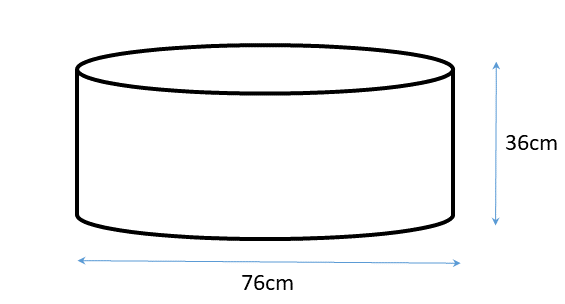It is filled at the rate of 0.3 litres per second. How long does it take to completely fill the tank?

[1 litre = 1000 cm3]

3.

GCSE Higher

Babatunde has to paint four containers.

Each container is in the shape of a cylinder with a diameter of 1.2m and a length of 3.2m.

How many tins of paint will Babatunde need to buy to completely cover each of the four containers if each tin of paint covers 6m2?

You must show all of your working.[The surface area of a cylinder of radius $$r$$ and height (or length) $$h$$ is $$2\pi rh + 2\pi r^2$$]

4.

GCSE Higher

A builder needs to lift a steel block. It is a cuboid with dimensions 2 m by 0.2 m by 0.2 m. Steel has a density of 7.6 g/cm3.

The builder's lifting gear can lift a maximum load of 500 kg. Can the lifting gear be used to lift the steel block?

5.

IGCSE Extended

[The surface area of a sphere of radius $$r$$ is $$4\pi r^2$$ and the volume is $$\frac43\pi r^3$$]

A solid metal sphere has a radius of 7.5 cm.

(a) Calculate the volume of the sphere to the nearest cubic centimetre.

(b) Calculate the surface area of the sphere to the nearest square centimetre.

(c) If one cubic centimetre of the metal has a mass of 4.9 grams calculate the mass of the sphere to three significant figures.

(d) Two of these spheres are placed in the water in a cylindrical tank with base diameter 32cm. Before they were lowered in the depth of the water was 19cm. Calculate the new depth of water in the cylinder when the spheres are fully submerged.

6.

GCSE Higher

A circular dart board has radius of 30 cm.

(a) Calculate the area of the front face of the dart board in cm2, giving your answer as a multiple of $$\pi$$.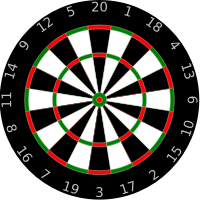(b) The volume of the dart board is 4500$$\pi$$ cm3. Calculate the thickness of the dart board.

7.

GCSE Higher

A batch of coins is made from an alloy consisting of 270g of copper mixed with 90g of nickel.

• The density of copper is 9.0g/cm3.
• The density of nickel is 8.9g/cm3.

(a) Work out the volume of copper used in the alloy.

(b) What is the density of the alloy to three significant figures?

8.

GCSE Higher

The diagram below shows two rectangles not drawn to scale.

The perimeter of rectangle EFGH is 41cm and the area of rectangle ABCD is 55cm2.

• EF = 9.5cm
• AB = FG9.

GCSE Higher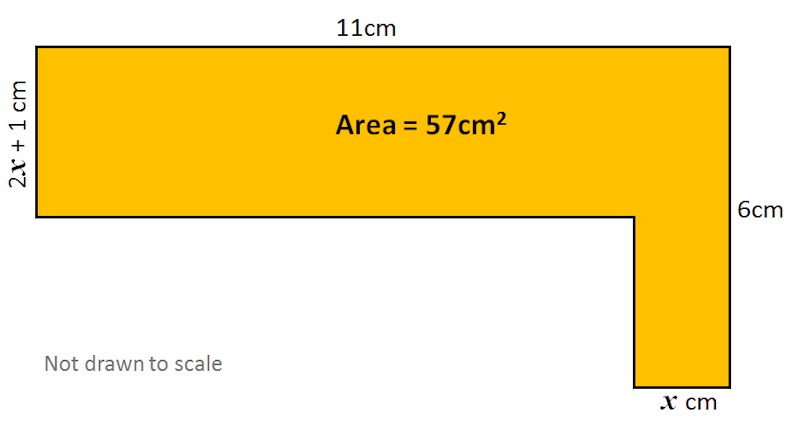Find the value of $$x$$.

10.

GCSE Higher

A yellow equilateral triangle has been painted on a purple sector. The side OC is 20cm and OA is 12cm. Calculate the area of the purple region ABCD as a percentage of the area of the whole sector OCD.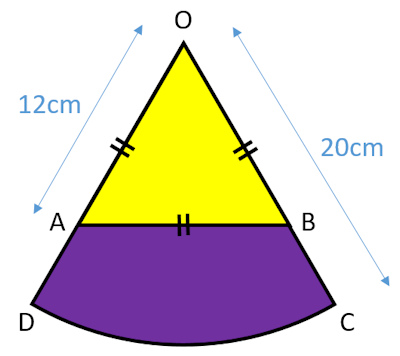The diagram is not drawn to scale.

11.

IGCSE ExtendedA circle is drawn inside a square so that it touches all four sides of the square.

(a) If the sides of the square are each $$k$$ mm in length and the area of the red shaded region is $$A$$ mm2 show that:

$$4A=4k^2-\pi k^2$$

(b) Make $$k$$ the subject of the formula $$4A=4k^2-\pi k^2$$

12.

GCSE Higher

Find the length of the sides of a square from the following clues:

• The square is drawn inside a circle.
• Each vertex of the square lies on the circumference of the circle.
• The area of the circle is 64cm2.

13.

IB Studies

Twenty four spherical shaped chocolates are arranged in a box in four rows and six columns.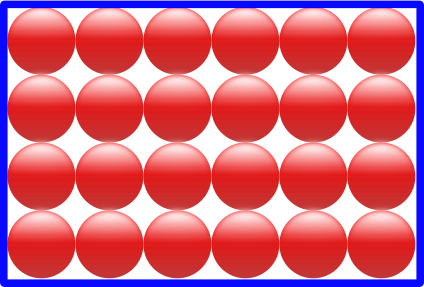Each chocolate has a radius of 1.2 cm.

(a) Find the volume of one chocolate.

(b) Write down the volume of 24 chocolates.

The 24 chocolates fit perfectly in the box with each chocolate touching the ones around it or the sides of the box.

(c) Calculate the volume of the box.

(d) Calculate the volume of empty space in the box.

(e) What percentage of the contents of the box is chocolate?

14.

IGCSE Extended

The three sides of an equilateral triangle are tangents to a circle of radius $$r$$ cm. The sides of the triangle are each 10cm long.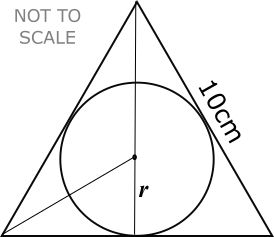(a) Calculate the value of $$r$$.

The second diagram shows a box in the shape of a triangular prism of length 15cm.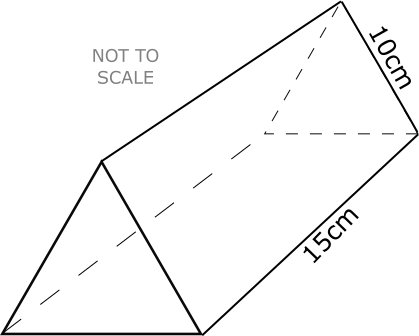The cross section is an equilateral triangle with sides of length 10cm.

(b) Calculate the volume of the box.

(c) Calculate the largest number of cookies that will fit in the box.

(d) Calculate the volume of one cookie in cubic centimetres.

(e) Calculate the percentage of the volume of the box not filled with cookies.

15.

GCSE Higher

A square has sides of length $$x$$ cm.

The equilateral triangle next to it has sides which are each 3cm more than the length of a side of the square.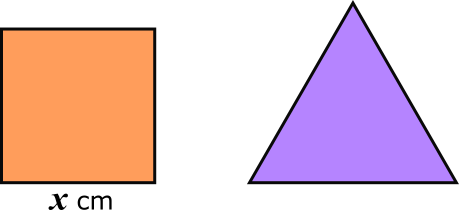(a) Find the perimeter of the square if it is equal to the perimeter of the triangle.The diagram above show the same square and triangle.

The length of the diagonal of the square is $$d$$ cm and the height of the triangle is $$h$$ cm.

(b) Which has the greater value, $$d$$ or $$h$$?

16.

GCSE Higher

The diagram, drawn to scale, shows a right-angled triangle ABC.Construct using a ruler and a pair of compasses a rectangle, DEFG, equal in area to the area of the triangle ABC and with DE the same length as AB.

You must show all your construction lines.

17.

GCSE Higher

Three crayons are held together with an elastic band. The diagram below shows the end of the crayons and the elastic band.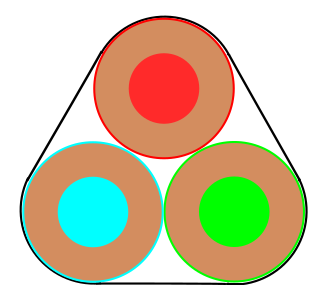Each of the crayons has diameter of 10 mm. Find the length of the elastic band in this position

18.

GCSE Higher

(a) Find the area of a regular octagon if the distance from its centre to any vertex is 10cm.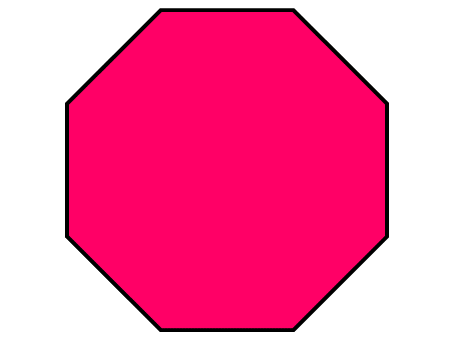(b) If the octagon had been cut from a piece of square card that was only just large enough, work out the area of the original square piece of card.

(c) A table top is made in the shape of a regular octagon with sides five times as long as the card model. Find the ratio of the area of the table top to the area of the card model.

19.

GCSE Higher

Four congruent triangles come together to make a square as shown in the diagram. The ratio of the area of one triangle to the area of the square is 1:5. Find the area of the square in square centimetres.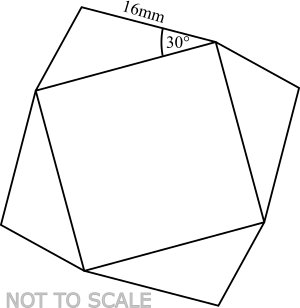20.

GCSE Higher

Five identical circles fit exactly inside a rectangle as shown in the diagram.

Find the area of the rectangle in terms of $$r$$, the radius of the circles.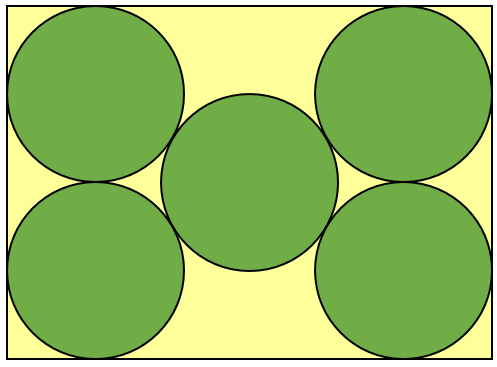21.

GCSE Higher

The volume of a cone can be calculated using the formula $$V=\frac13 \pi r^2 h$$ and the area of the curved surface of a cone can be calculated using $$A= \pi r l$$ (where $$r$$ is the radius and $$l$$ is the slant height).

(a) Calculate the volume of this frustum;

(b) Calculate the total surface area of this frustum.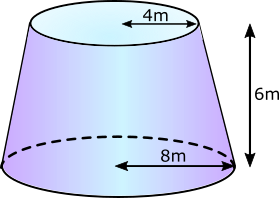22.

GCSE Higher

Harper mixes 300g of material $$X$$ and 150g of material $$Y$$ to make 450g of a compound material.

Material $$X$$ has a density of 20g/cm3.

Material $$Y$$ has a density of 15g/cm3.

Work out the density of the compound material.

23.

GCSE Higher

The diagram shows a water tank in the shape of a trapezoidal prism.Winthrop begins filling the tank with a hose pipe. After 30 minutes there are 900 litres of water in the tank. How many more minutes will it take until the tank is half full? ( $$1m^3 = 1000$$ litres )

24.

IB Analysis and Approaches

A metal sphere has a radius 7.2 cm.

(a) Find the volume of the sphere giving your answer in standard form.

The sphere is to be melted down and remoulded into the shape of a square-based pyramid with a height of 10.3 cm.

(b) Find the length of one side of the pyramid's square base to the nearest millimetre.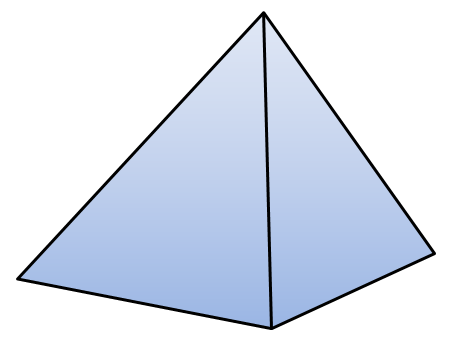25.

IB Studies

A solid metal cylinder has a base radius of 5cm and a height of 9cm.

(a) Find the area of the base of the cylinder.

(b) Find the volume of the metal used in the cylinder.

(c) Find the total surface area of the cylinder.

The cylinder was melted and recast into a solid cone with a circular base radius, OB (where O is the centre of the circle), of 7cm. The vertex of the cone is the point C.

(d) Find the height, OC, of the cone.

(e) Find the size of angle BCO.

(f) Find the slant height, CB.

(g) Find the total surface area of the cone.

If you would like space on the right of the question to write out the solution try this Thinning Feature. It will collapse the text into the left half of your screen but large diagrams will remain unchanged.

The exam-style questions appearing on this site are based on those set in previous examinations (or sample assessment papers for future examinations) by the major examination boards. The wording, diagrams and figures used in these questions have been changed from the originals so that students can have fresh, relevant problem solving practice even if they have previously worked through the related exam paper.

The solutions to the questions on this website are only available to those who have a Transum Subscription.

Exam-Style Questions Main Page

Search for exam-style questions containing a particular word or phrase:

To search the entire Transum website use the search box in the grey area below.